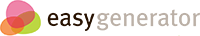# Learning How to Solve for "x"

## Frequently asked questions involve, "Should I add before I subtract?" or "Do I calculate what is in the parenthesis first?## We will demonstrate how to solve for a missing variable with the use of orders of operations

### Self-Check: What are the steps of Order of Operations

• Step 1
Parenthesis
• Step 2
Exponents
• Step 3
Multiplication
• Step 4
Division
• Step 5
• Step 6
Subtraction

### Applying the Order of Operations

Please solve for the Missing Variable using the Order of Operations.

(4-3)X = (32÷2)÷4

Step 1.  X = (32÷2)÷4

Step 2.  X = ÷4

Step 3.  X =